# Optimal Juggling

### The Analysis and Over-analysis of Juggling Patterns

#### Introduction

Juggling has been one of the greatest pleasures in my life, since 1980 when I taught myself to juggle three balls. After receiving a B.S. in Mechanical Engineering from Carnegie Mellon University, I was able to combine my interests in juggling and engineering while working for IBM Research on a juggling robot. Since then, I have become a professional entertainer, but I have continued to pursue my interest in engineering and mathematics in my spare time. This paper is a result of that pursuit.

The mathematics of juggling is arguably a frivolous field of research, even more so than juggling itself. However, I have found exciting results about what ideal or spatially optimal juggling patterns should look like. I believe these results can help jugglers improve their patterns.

I define a spatially optimal pattern as one that allows for the same amount of error at all points along the flight path where collisions are most likely. This is based on the principle that a chain is only as strong as its weakest link. If a juggler has an inefficient pattern with much more room for error in one area than in another, the juggler may be wasting time and energy.

Although jugglers have notoriously tossed objects including clubs, rings, kittens, and chainsaws, this paper will only discuss balls, which are the most common juggling objects and the easiest to analyze. I will also limit discussion to ideal patterns, which are strictly two-dimensional and have no error.

Juggling patterns have two parts: ball motion and hand motion. I will primarily discuss juggling from the point of view of the balls. Hand motion must correspond to the positions and velocities of the balls during throws and catches, but I will not analyze this motion in-depth because accurately modeling the dynamics of a human arm would be next to impossible.

I believe that the hands must conform to the juggling pattern much like an expert typist's hands conform to the keyboard layout. Jugglers may have personal preferences for how fast and how high they feel comfortable throwing, but the other parameters, such as how far to throw and how far to move your hands, can still be optimized.

I believe that by practicing, a juggler's patterns evolve by trial and error, approaching spatially optimized patterns. Like natural selection, bad patterns die early while good patterns live longer and get reinforced. I have observed that after many hours of trial and error practicing, the best jugglers have patterns that are spatially optimized. I think that jugglers could speed this learning process and improve their form by seeing what these optimal patterns looks like before starting.

I have created a computer program called Optimal Juggler (only for Macintosh) which displays the optimal patterns described in this paper. The user can adjust many of the pattern variables to suit personal preferences, and the optimal pattern is instantly computed and displayed in real time. I highly suggest having a copy of this program while you read this paper. I have made the program available for free on the Internet from the Juggling Information Service at http://www.juggling.org/.

#### The Physics of Juggling

I will introduce several equations regarding the timing of juggling patterns. First, I must define some parameters. In all juggling patterns, thrown balls follow parabolic arcs. The word arc is used throughout this paper to refer to the path of a ball in flight, from a throw to a catch. Some universal parameters for juggling are:
b = number of balls
h = number of hands
f = flight time (the time a ball is in the air between throw and catch)
g = acceleration due to gravity (~ 9.81 meters / second / second)
Vh = horizontal velocity of throw
Vv = initial vertical velocity of throw
H = throw height
F = flight distance (horizontal distance of a ball flight)

Claude Shannon, who is best known for his contributions to the field of mathematics and computer science, was also one of the first to explore the mathematics of juggling. He defined two variables in his work that I haven't listed above:
d = dwell time between a catch and the next throw
e = empty time between a throw and the next catch

Shannon's juggling theorem elegantly relates the variables of the pattern's timing with the number of objects being juggled and the number of hands:
b / h = (d + f) / (d + e) .

Instead of referring to d and e in my work, I have found it useful to incorporate these quantities into some new variables:
tau = hand period or time between two throws from the same hand. This is the same as d + e.
r = "dwell ratio" or the fraction of time a hand is full. This is d / tau , or d / (d + e).
omega = the average number of balls in flight per arc. This is f / tau , or f / (d + e).

The defining equation for omega can be rewritten:
tau = f / omega , or f = tau * omega.

Note that the dwell ratio, r, is always between 0 and 1, and can also be described as the average number of balls occupying a hand while juggling.

Substituting these new variables into Shannon's equation yields:
b / h = omega + r , or omega = (b / h) - r.
This is an important equation, which I will use throughout this paper.

When looking at a juggler, omega can be found by looking at the balls in the air. But if we don't also look at the hands, it may be impossible to determine exactly how many balls are being juggled. For example, if we see a juggler with two arcs of balls in the air and omega = 1.25, it is not clear whether he is juggling three or four balls. To determine whether it is three or four, we need to look at the dwell ratio of the juggler's hands, which could be holding between zero and two balls.

By definition, juggling requires at least one more ball than there are hands. So, with 2 hands, omega has a minimum value of 0.5, when b = 3 and r = 1.

Newtonian physics provides some equations that apply to thrown objects:
Vh = F / f
Vv = sqrt(2 * g * H)
H = (Vv ^ 2) / 2 / g

If the throws and catches are assumed to occur at the same height, we can solve for the flight time, f, and substitute it into the other equations. As a result, we get some useful equations:
f = sqrt(8 * H / g)
Vv = g * f / 2
H = g * (f^2) / 2 = g * [(tau * omega)^2] / 8

These equations tell us that to double the flight time, you must double the initial throw velocity which means quadrupling the throw height.

##### Graph 1: Throw Height as a Function of tau and omega (assuming throw and catch positions at the same height, g = 9.81 m/s/s)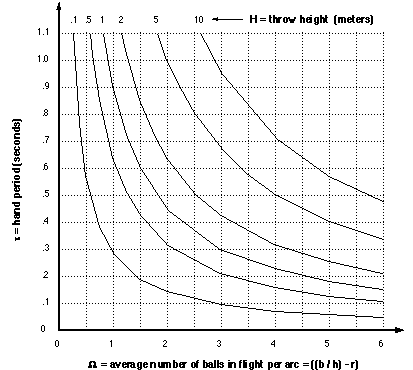In the introduction, I mentioned that this paper would only discuss ideal patterns with no error. But in reality, even the most skilled juggler must allow for some amount of error. All throws in a real juggling pattern will have some error in the position and velocity in all three dimensions, as well as time.

In order to write some equations, I will define throw position errors: dx, dy, and dz, throw velocity errors: dvx, dvy, and dvz, and throw time error: dt. I determine how the different throw errors affect the ball flight by comparing the positions of a ball thrown properly and a ball thrown with each of the errors.

##### Error in Ball Position at Time = t After Throw
 x error y error z error Error in Throw Position dx dy dz Error in Throw Velocity dvx * t dvy * t dvz * t Error in Throw Time dt * Vh dt * [Vv - (g * t) + (dt *g / 2)] 0 TOTAL ERROR at Time = t dx + (dvx * t) + (dt * Vh) dy + (dvy * t) + {dt * [Vv - (g * t) + (dt *g / 2)]} dz + (dvz * t)

Looking at the equations, it seems these errors in ball position can be decreased by reducing the flight time, throw velocity, and the horizontal and vertical dimensions of the arcs. In practice, reducing the height of a pattern can decrease error and make a pattern more stable. Unfortunately, this also reduces the time to make each throw, which may make it harder to make accurate throws.

On the other hand, errors can sometimes be reduced by loosening the temporal constraints on the hands. Allowing more time for each throw often results in better throws even if it means increasing the height of the pattern. Jugglers must compromise between having more time and having better accuracy, to find the most comfortable and stable pattern dimensions.

Jugglers generally position their hands comfortably in front of (and slightly below) their shoulders. Since this position is near the middle of the hands' range of reach, it also allows for a lot of flexibility in catching inaccurate throws. We would therefore expect the distance between the arcs in a fountain, and the flight distance in a cascade, to be roughly equivalent to the width of the juggler's shoulders. Data taken from jugglers on videotape supports this hypothesis.

Adjusting for error can be very complicated. Error tends to propagate. For example, after catching a ball with a large error in position, the next throw is likely to be worse than if the catch had been good. A skillful juggler is able to maintain a stable pattern by keeping these errors from growing out of control. Good jugglers will often accommodate a bad throw by adjusting the tempo and direction of subsequent throws, and often throwing balls up wherever they see open space. Catches can also be made slightly sooner or later than the predicted time by intercepting the ball at a different point on the arc. Skillful jugglers can also make corrections in the throwing velocity and angle, so that even if a ball must be thrown from a bad position, it may be caught at a good position.

Accurately modeling error would be very difficult. From a statistical analysis of a juggler with a given pattern, an error zone around each ball could be determined, where the balls have some probability of being inside the error zone. The error zone around each ball is a fuzzy area which probably changes size and shape during the ball flight.

In the upcoming analysis, a constant circular error zone around each ball can be modeled by simply adding a safety factor into the diameter of the balls (using a larger value for the ball diameter than the actual diameter of the ball). Please note that a constant circular error zone is only an approximation.

#### Optimal Arcs

Each arc has some average number of balls, omega, that occupy it at any given time. Define an optimal arc as an arc which has the minimum dimensions that can accommodate that number of balls. When the minimum dimensions are achieved, each ball will touch the previously thrown ball at some point on the arc. (Notice that every ball will actually touch two other balls: the ball before it and the ball after it.) The ball size is now important so we must define a new variable:
D = diameter of the ball

Since the horizontal velocity of thrown balls remains constant, the horizontal distance between balls on the same arc is also constant. Observe two balls thrown consecutively at times t and t + tau. The balls are closest near the top of the arc when they are at the same height, one going up, one coming down.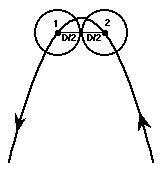Optimally, the two balls just graze at this point, so the horizontal distance between their centers is equal to one ball diameter. Since we know this horizontal distance is constant, we can write two simple equations, which I will call the optimal arc equations, relating flight distance, velocity, and timing with the size and the average number of balls that occupy an optimal arc:
F = omega * D
Vh = D / tau

Notice that these equations are independent of the height of the throws. Also notice the optimal arc must contain the apex of the parabola and extend a minimum horizontal distance of D/2 in each direction because the equations were derived from the fact that two balls could be on the same arc at the same height.

In theory, it is possible to have an arc with F < (omega * D) if the arc does not contain the apex of the parabola and extend a minimum horizontal distance of D/2 in each direction. But, patterns with this type of arc are of very little practical use because they would involve much more hand motion and speed than other patterns.

In a three ball pattern with r > 0.5, there is never more than one ball per arc (omega < 1). In this case, balls can never graze during an arc, so the optimal arc equations do not apply.

The cascade is the most commonly practiced juggling pattern. In this pattern, an odd number of balls is juggled between the two hands. The hands alternate throwing in an even rhythm along two symmetrical arcs. Each hand throws balls up near the middle of the pattern so that they cross and are caught near the outside of the pattern by the opposite hand. Throughout this paper the diagrams of juggling patterns have throw and catch positions labeled T and C. The path of the hand between these positions is represented by a dotted line; the exact path is unspecified.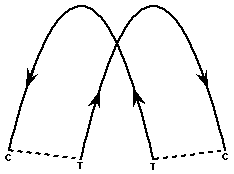Only a few jugglers have ever reached the level of cascading nine balls. Anthony Gatto is reported to have achieved runs of 30 seconds in practice.

If this pattern is done in reverse, throwing on the outside and catching on the inside, it is known as a reverse cascade. It is slightly harder than a normal cascade because the crossing point in the pattern comes later in the ball flight. This allows error to compound for a longer time before the crossing point where collisions are most likely.

#### Finding the Minimum Distance Between Two Arcs of a Cascade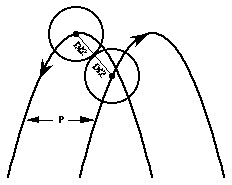Define a new variable:
P = horizontal distance between two parabolic arcs.

Assume we are juggling balls, with diameter = D, in a cascade pattern with the two arcs spaced a distance, P, apart. Look at two consecutively thrown balls, one on each arc. Assuming they graze each other in this manner, one can work backwards to find the optimal value of P.

Observe the two balls when they just touch. The motion of the balls can be most easily described at this point if we use the frame of reference of the lower ball. In this accelerating frame of reference, the lower ball is stationary and the upper ball has a constant velocity relative to the lower ball.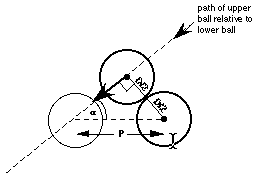Since the balls are traveling in opposite directions horizontally, the relative horizontal velocity is 2 * Vh. Vertically, the balls have the same acceleration, g, but were thrown at time difference, tau / 2 so the relative vertical velocity is g * tau / 2. After they touch, the balls continue moving until they are at the same height. Since the balls are on equivalent parabolas spaced P apart, the horizontal distance between the two balls at this point is equal to P.

One equation for tan(a) can be constructed from the distances using simple trigonometry:
tan(a) = D * (P^2 - D^2)^(-1/2) = [(P / D) ^2 -1]^(-1/2) .

Another equation for tan(a) can be written using the relative velocities:
tan(a) = (relative vertical velocity / relative horizontal velocity) = g * tau / 4 / Vh .

Set these results equal to each other, and solve for (P/D). The result is:
(P/D) = [(4 * Vh / g / tau)^2 +1]^(1/2) .

If we assume that we are using optimal arcs, we can substitute the optimal arc equations into this equation for P. The result is that P can be expressed solely as a function of hand period, tau , and ball diameter, D, since gravity, g, is constant:
(P/D) = [(4 * D / g / tau / tau)^2 +1]^(1/2) .

There are several important things to notice about these results:

1. This optimal value for P is independent of the number of balls, the endpoints of the arcs, etc. The endpoints of the arc (the throw and catch positions) are determined by the average number of balls that must occupy the arc. Omega increases as the endpoints are moved downward to include more of the arcs.

2. P/D is always greater than 1. For human jugglers performing a cascade, the optimal value of P/D is generally very close to 1. In fact, an optimal cascade with P/D > 1.1 is very difficult because it would require juggling large balls very fast.

The optimal distance between the arcs of a cascade is always approximately one ball diameter; therefore even in a cascade with very large numbers of balls, the hands may only need to move a short distance. This is an advantage that not all patterns have, as we will see later.

The shape and relative positions of the arcs are defined by the equations for P and the optimal arc equations. We now must determine the optimal endpoints of the arcs, which will be the throw and catch positions. We will not assume throws and catches are at the same height. Define the following new variables:
E = dwell distance = distance between catch position and throw position
theta = dwell angle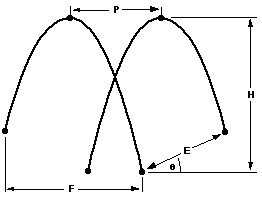Assume we are given two parabolas in a cascade, and the values for Vh, F, and P are also given. We would like to find the exact throw and catch positions on these parabolas that minimizes the distance between throw and catch. (The equations start to get messy here, so I'll just summarize the method and the results.) First, find the optimal value of theta to minimize the dwell distance, E. This is done by finding an equation for E in terms of the given quantities and theta, and setting the derivative with respect to theta, dE/d(theta) = 0. The result is:
tan(theta) = 2 * Vh * Vh / g / F .

Substituting this value back into equations for E and H, we get the following results:
E = P * cos(theta) .
This means the distance the hands must move, E, is slightly less than P but E is still always greater than the diameter of a ball, D. The result for H is:
H = {F + [P * sin(theta) * sin(theta)]}^2 / [4 * F * tan(theta)]
As mentioned earlier, if theta is small, H can be approximated with:
H = g * f * f / 8 .

There is a limit to how low you can make an optimal cascade. The balls from one arc must graze the balls from the other arc. The limit is reached when, at the instant each ball is thrown, it grazes the previously thrown ball from the other hand. Here is a summary of how to find the equation for this limit. First, write an equation for the distance between two consecutive throws from opposite hands. By setting the time derivative of this distance = 0, we find the time at which the distance between the balls is minimized and, in an optimal pattern, they are touching. By setting this time = 0, we get an equation for patterns where balls are grazing at the instant of the throw. The result, included for completeness, is: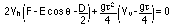For all spatially optimal cascades with five or more balls, the equation for P and the optimal arc equations will both be applied. Each ball will touch four other balls during the flight: the balls before and after it on the same arc, and the two balls that it passes between on the other arc. In an optimal cascade with three or five balls, each ball grazes every other ball in the pattern.

In the case of a cascade with three balls, there are only two other balls. Since any two balls in a three ball cascade are consecutive, if two balls graze anywhere then every ball grazes every other ball, so the pattern is spatially optimized. The equation for P is the only equation necessary to define a spatially optimized three ball cascade. The optimal arc equation can be applied to three ball cascades with omega >= 1, but it is an unnecessary constraint.

All of these results for spatially optimal cascades are displayed in the companion computer program to this paper, Optimal Juggler (only for Macintosh).

#### The Best Dwell Ratio

What is the best value of r? After a ball is caught, the hand must move from the catch position to the throw position. And after the ball is thrown, the hand must move the same distance back to the catch position. Therefore, a reasonable initial assumption would be that r = 0.5. However, there are several reasons why a greater dwell time is advantageous:

1. The hand velocity must match that of the ball while throwing. But to catch a ball, we only need to match the position accurately, since we can grip the ball when it hits the hand. Because throwing is more constrained than catching, r should be greater than 0.5.

2. The weight of the balls can affect the hand motion. In cases where the weight of the balls is not negligible, more effort is required during the dwell time to absorb the momentum of the ball being caught and to impart the momentum of the following throw. Therefore, to help minimize hand forces when juggling heavier objects, increase the dwell ratio, r.

3. Increasing r gives the juggler more time to control the ball and therefore, greater accuracy.

4. Increasing r also decreases omega which can slightly lower the chance of collisions.

Therefore, the best dwell ratio is probably somewhere between 0.5 and 1. The exact value is determined by how the juggler compromises throwing accuracy and catching ease. If the dwell ratio is decreased, less time is available to make accurate throws. If the dwell ratio is too high, catching may be more difficult since less time is available to get to the catch position. Various tests with highly skilled jugglers show that the preferred dwell ratio for a cascade with 3, 5, 7, or 9 balls is near r = 2/3 .

The following patterns are of little practical use because they can only be done by juggling infinitely fast or where g=0. Although I will not explore them in depth, there are some interesting examples:

The pattern height can be made arbitrarily small if the arcs do not intersect. If H is lowered to zero, the arcs become horizontal lines. A pattern of this type with five balls and r = 0 would look like this: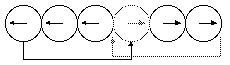With three balls, if omega < 1, the pattern dimensions can be completely minimized, with the arcs actually reduced to points. F = 0, H = 0, E = D, Vh = 0, Vv = 0.

With five or more balls, F can be reduced to zero if throws are made vertically in two columns. Each ball is caught at the top of one column and then thrown up in the other column. The columns can be spaced a minimum distance of P = D / sqrt(2). With five balls and r = 0, the pattern looks like this: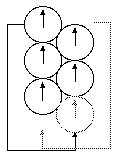#### The Fountain

For juggling an even number of balls, the fountain pattern is the most common. The two hands juggle independent patterns, with half of the balls in each hand. Throws are usually synchronized or 180 degrees out of phase. Like the cascade, both hands throw near the middle and catch at the outside of the pattern.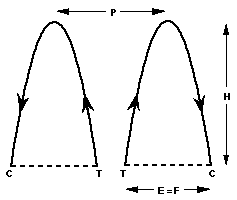Since the same hand must catch the balls that it throws, E = F. To minimize throw and move distances, the throws and catches should be made at the same height. The optimal arc equation also applies to all optimal fountains:
E = F = omega * D .

Notice that the distance the hands move in a fountain increases, proportional to the number of balls, without a finite limit. Therefore, the fountain has a disadvantage for juggling large numbers of balls.

The height of a fountain is independent of all other pattern dimensions and can be made arbitrarily small. The best height depends on the preferred value for tau:
H = g * [(tau * omega)^2] / 8 .

Since the hands are juggling completely independently, the value of P is independent of all other pattern parameters. P tends to be approximately the width of the shoulders.

Because the throws in the fountain are aimed toward the outside of the pattern (unlike the cascade where throws are made towards the middle) the hand must reverse the horizontal velocity of the ball twice (in a cascade the hand reverses the horizontal velocity of the ball only once). Between a throw and the next catch, the hand does not need to reverse horizontal direction at all. Since a more complex motion must be done between catch and throw, the dwell ratio, r, would be expected to be greater than .5, and would even be expected to be higher than in a cascade. However, in my observations, the preferred dwell ratio for fountains with 4, 6, and 8 balls is near r = 2/3, about the same as cascades.

Few jugglers have been able to juggle eight balls in a fountain pattern for more than a few cycles.

#### Asymmetrical Crossing Patterns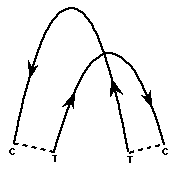There is a wide range of possible asymmetrical crossing patterns, with different timings and height ratios. The timing can range from synchronized to 180 degrees out of phase, and the hands can throw to the same height or vastly different heights, as long as you can avoid collisions where the balls cross.

For large, even numbers of balls, many jugglers prefer a synchronized pattern like this where one hand throws slightly higher than the other to avoid collisions in the middle. This pattern has an advantage over the fountain because it requires less hand motion. If the juggler has a dominant hand, he may vary the heights more so that the dominant hand does most of the work, while the non-dominant hand does less work.

Asymmetrical crossing patterns are also possible for odd numbers of balls, but this is rarely seen since the cascade is generally easier.

Since the hands can have different motions and different dwell ratios, these added variables would make finding the optimal pattern much more difficult than it is for cascades and fountains.

Few jugglers have been able to juggle eight balls in this pattern for more than a few cycles. Bruce Sarafian has reportedly achieved 20 catches with 10.

#### The Half Shower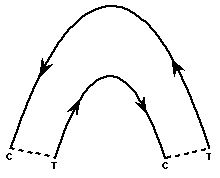The half shower pattern is asymmetrical, but the crossing point is eliminated. One hand makes long, high throws over the top, and the other hand makes shorter, lower throws under it. This pattern is commonly done with both odd and even numbers of balls. One hand does more of the work, which can be advantageous for jugglers with a dominant hand. The distance between throw and catch can be even smaller than the cascade.

With different numbers of balls, the height ratio or the timing must also change. As with other asymmetrical patterns, the variable heights and timings make finding the optimal pattern very difficult.

A theoretical optimal half shower (possible if g = 0 or juggling infinitely fast), would look like this (with 8 balls):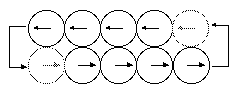#### The Shower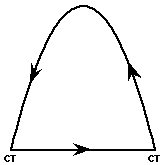In a shower, one hand throws the balls in a high arc over to the other hand and the second hand passes the balls very quickly back to the first hand. This pattern can be used for any number of balls, and the timing can remain constant. The dwell distances can be made very small. The hands may have different dwell ratios.

The height and speed of the high throws in a shower with (b) balls is about the same as in a cascade with (2 * b - 1) balls. Since one hand is doing almost all of the work of keeping balls in the air, it is very difficult to shower a large number of balls. Few people have been able to successfully shower seven balls for more than one cycle.

#### Columns

Another interesting pattern for one hand is the columns pattern. Each ball occupies one column and is thrown straight up while the hand moves from column to column, catching and throwing.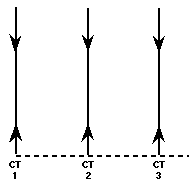Optimally in the columns pattern, F = 0 and the horizontal throwing and catching velocities = 0. There is no reason for the columns to be more than the minimum distance of one ball diameter, D, apart. This makes moving the hand between columns as easy as possible. Throw height, H, depends on preferred throwing speed, and can be made arbitrarily low.

If two balls are being juggled in one hand, the hand just alternates between the two. With more than two balls, there becomes more than one possible order to visit the columns. The hand could start at one end and move across to each column (1,2,3,4,...) and then have one long move at the end of the cycle back to the first column. A better pattern would probably be to start at one end throwing in the odd columns (1,3,5,...) and come back throwing in the even columns (..., 6,4,2). This would have the minimum total hand motion possible and no single move of longer than 2 columns. The average move distance over the whole hand cycle = [2 - (2 / b)] * D .

In the cascade and the fountain, the hand moves two times the distance, E, for every throw because it has to move from the throw position to the catch position and then back again. In the columns pattern, there is only the move from a throw to the next catch. There is no horizontal movement between a catch and the next throw. As the number of balls is increased, the average distance between throws in the columns pattern approaches a finite limit of 2 * D.

In theory, making pattern three-dimensional and arranging the columns in a horizontal loop would eliminate moves longer than 1 ball diameter.

In practice, I have never seen jugglers use the columns pattern with more than three balls in a hand.

#### Bounce Juggling

If juggling rubber balls over a hard floor, it is possible to do any of the previously described patterns by bouncing the balls off the floor. Bounce juggling is most commonly performed with a cascade.Bounce juggling requires less physical effort because the balls are not thrown very high. Less hand motion is necessary because dwell distances can be very small and the balls are thrown at slower speeds. The balls are easier to catch because their velocity is slowest at the top of the pattern. A disadvantage is that, when juggling lots of balls, it may be necessary to stand on a chair, etc. to gain more time. Also, mistakes often result in catastrophic collisions that scatter bouncing balls around the room.

All of the equations for the optimal cascade apply to a bounce cascade - until the point of the bounce. Since no balls bounce perfectly, a complete analysis of anything after the bounce is very difficult.

The endurance record for bouncing seven balls is over 20 minutes by Pat McGuire. Ten balls has been controlled for a few cycles in a crossing pattern by Tim Nolan.

#### Flashing

The word juggling implies a continuous pattern where each time an object is caught, it is thrown back up again. If a juggler throws all the balls up once in a pattern and then catches them all, it is commonly called a flash. From the point of view of the balls in the air, flashing is the same as juggling.

Unlike continuous juggling, however, the hand movement while flashing does not have to alternate between the catch position and the throw position. For example, when flashing a cascade or fountain, the dwell distance, E, is only traveled once, after all the balls are thrown and before they are caught. This makes flashing considerably easier than juggling.

More hand motion is required for flashing columns, where the hands must still move a distance of D between throws.

Eleven balls has been flashed in a cascade by Bruce Tiemann and Bruce Sarafian. Twelve balls is the largest number to be flashed, in an asymmetrical crossing pattern by Bruce Sarafian.

#### Conclusions

Juggling is constrained by the spatial limits of the objects in the air. The size of the objects, juggler's hand range, and throw accuracy all limit the juggler spatially. However, describing spatially optimal patterns is only the first step in determining how one should juggle. One must also consider the temporal constraints on the hands and the throw errors that accompany them to find the most comfortable juggling speed.

Which pattern is easiest? The answer is mainly determined by which pattern the juggler has practiced. Generally, the easiest patterns are the ones where both hands are working equally and all the throws are the same. As mentioned earlier, patterns where one hand is throwing higher than the other are less efficient at keeping balls in the air, and result in higher or faster juggling, than patterns where both hands work equally. Juggling the balls in a cascade, fountain or columns would all result in approximately the same height of pattern. Vertical hand motions would also be roughly the same for the three patterns. For large numbers of objects, the cascade has an advantage with far less horizontal hand motion than the fountain. Columns also has more hand motion than the cascade and the disadvantage that throws are made from different places. Therefore, to juggle a large number of balls, I recommend practicing the cascade.

Further evidence of the cascade's advantage over the fountain can be seen in data taken from one of the world's best jugglers, Anthony Gatto. He actually has more room for error in his 9 ball cascade than he does in his 8 ball fountain. His dwell distance with the 8 ball fountain was about 0.44m, while with a 9 ball cascade, it was only 0.34m. Using the equations I have derived, it is possible to work backwards to estimate the allowable error in any juggler's pattern. With the 9 ball cascade, Anthony Gatto had a ball safety zone diameter of about 0.20m. With the 8 ball fountain, it was only about 0.13 m.

The following advice regarding correcting collision problems is derived from the research in this paper. With more than three balls, collisions generally happen so fast that it is difficult to determine what exactly went wrong. There are two places where collisions are most likely to occur: collisions between consecutively thrown balls from the same hand, or between consecutively thrown balls on different arcs.

If you consistently get collisions near the top of an arc between balls on the same arc, try increasing the flight distance, F. If using a fountain pattern, this also means increasing dwell distance, E.

If you get collisions between balls near the crossing point in a cascade, try increasing E.

When trying to change any parameter, it may help to slow the pattern down by also increasing height, H. Also increasing the dwell ratio, r, can help because it slightly decreases the number of balls in the air, and gives you more time to aim your throws.

As I mentioned earlier, I have found that the best jugglers in the world develop, by trial and error , patterns which are very close to the optimal patterns described in this paper. If you want to improve your juggling form, I recommend that you get a copy of my computer program Optimal Juggler (for Macintosh).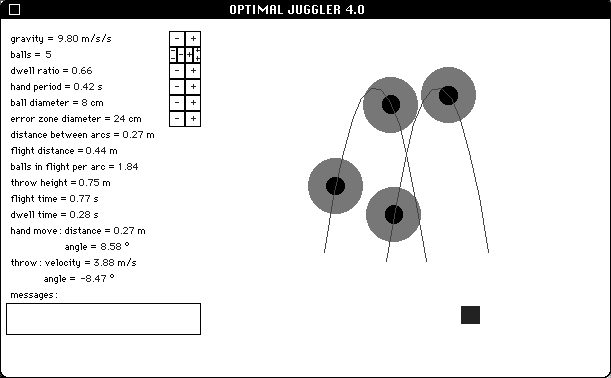Above is a picture of the actual Optimal Juggler program window. The program allows you to easily modify several parameters of the animated juggling pattern to match your preferred style, and displays what your optimal pattern should look like in actual speed or slow motion. Actually seeing what the optimal pattern looks like is very helpful in the learning process.

#### Index - Tables of Preferred Juggling Parameters

The following table of preferred hand periods was measured live or from video. All data was taken from what appeared to be stable, comfortable patterns. (Frequencies should never be measured during a performance, especially to music, since the rhythm of the music may affect the juggler's speed.) All jugglers used cascades for odd numbers and fountains for even numbers unless otherwise noted.
 # balls (jugglers using preferred objects - size & weight vary) preferred tau (seconds) 3 0.47- R. Rubenstein (1994) 0.54 - J. Kalvan (1994) 0.62 - B. Olson (1994) 4 0.53 - J. Kalvan (1994) 5 0.46 - R. Rubenstein (1994) 0.49 - J. Kalvan (1994) 0.50 - B. Olson (1994) 6 0.47 - J. Kalvan (1994) 7 .40 - D. Bennett (1984) 0.44 - D. Tison (1987) 0.44 - A. Gatto (1989) 8 0.31* - D. Bennett (1984) 0.38 - A. Gatto (1989) 9 0.30 - D. Bennett (1984) 0.33 - A. Gatto (1989)

* crossing 8 ball pattern.

The following average measurements were taken from videotapes:
# balls H E F tau r
3 (D = 7.5 cm) (Jack Kalvan) 0.33 m 0.20 m 0.33 m 0.55 s ~0.63
4 (D = 7.5 cm) (Jack Kalvan) 0.65 m 0.25 m 0.25 m 0.53 s ~0.68
5 (D = 7.5 cm) (Jack Kalvan) 1.0 m 0.24 m 0.39 m 0.47 s ~0.68
6 (D = 7.5 cm) (Jack Kalvan) 1.5 m 0.38 m 0.38 m 0.47 s ~0.71
7 (D = 7.5 cm) (Jack Kalvan) 1.8 m 0.47 m 0.48 m 0.42 s ~0.69
7 (D = 6 cm) (Anthony Gatto) 1.7 m 0.37 m 0.65 m 0.44 s ~0.68
8 (D = 6 cm) (Anthony Gatto) 2.2 m 0.44 m 0.44 m 0.38 s ~0.63
9 (D = 6 cm) (Anthony Gatto) 2.2 m 0.34 m 0.68 m 0.33 s ~0.63

#### References

Airborne! - Highlights of the 1989 International Jugglers Association Convention (1989), New York: IJA / Maverick Media

Beek, Peter Jan (1989), Juggling Dynamics, Amsterdam: Free University Press.

Buhler, Joe; Graham, Ron (1984), Fountains, Showers, and Cascades, The Sciences, vol. 24, pp. 44-51.

Donner, Marc D.; Kalvan, Jack (1988), Recent Progress in Juggling Robot Research, IBM Research Report, Yorktown Heights, NY.

Lewbel, Arthur (1987-94), The Academic Juggler, Juggler's World:
Memorable Tricks and a Numbers Formula, Winter 1987-88, p. 31.
A Short Lesson in Thought and Gravity, Summer 1988, p. 31.

Magnussson, Bengt; Tiemann, Bruce (1989), The Physics of Juggling, The Physics Teacher, pp. 584-588.

Shannon, Claude E. (1993) Scientific Aspects of Juggling in Claude Elwood Shannon Collected Papers, edited by N. J. A. Sloan and A. D. Wyner, New York: IEEE Press. pp. 850-864.

Optimal Juggling / Juggling Papers / jis@juggling.org